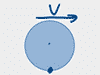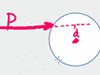21. # Forces (F=ma)

27. ### Elevator Force & Acceleration Problems: scale weight, acceleration

#### The videos in this chapter cover word problems about elevators, specifically the ones where they tell you how fast the elevator is accelerating up or down and then ask you how much the scale under the passenger would say the person weighs. Examples covered:33. ### Centripetal Force - Objects Swung In Circles: yo-yo's, conical pendulum, mass on string, breaking strength, slack velocity

#### The videos in this chapter cover problems where a mass on a string is being swung around in a circle. Could be a yo-yo, getting whipped in a vertical circle or a horizontal circle around someone's head. Could be a mass on a frictionless table with a weight on the string in a hole in the middle of the table. In each problem, you'll be asked to calculate either the velocity or radius of the circle which would: cause the string to go slack, break the string, or achieve equilibrium. Examples covered: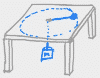34. ### Centripetal Force Problems - Cars, Planes & Roller Coasters: banked turns, normal force, coefficients of friction, car leaving the road/track

#### These videos cover lots of examples of centripetal force problems where the object going in a circle is a vehicle. In the case of roller coasters and jets (and some car problems), the circle is usually vertical and you're being asked to find the speed at which the person in the vehicle is "weightless" at the top of the loop (i.e. normal force is zero). In other car problems, the circle is horizontal (a curve in the road), and you're being asked how high to bank the turn or what coefficient of friction would prevent the car from sliding off the road. Examples covered: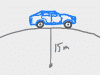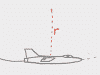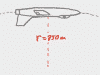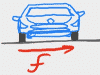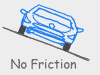41. # Rotational Motion

50. ### Rolling Motion

#### Rolling is more complicated than you'd think! On the one hand, you've got a mass on the move, so all the usual linear kinematics and energy formulas work. On the other hand, anything that is rolling is also rotating, which means it's got rotational kinetic energy too. It gets a bit complicated, but it's kind of cool if you study it because our lives are full of wheels: